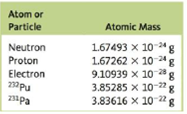# Many transuranium elements, such as plutonium-232, have very short half-lives. (For 232 Pu, the half-life is 36 minutes.) However, some, like protactinium-231 (half-life = 3.34 × 10 4 years), have relatively long half-lives. Use the masses given in the following table to calculate the change in energy when 1 mole of 232 Pu nuclei and I mole of 231 Pa nuclei are each formed from their respective number of protons and neutrons. Atom or Particle Atomic Mass Neutron 1.67493 X 10 -24 g Proton 1.67262 X 10 -24 g Electron 9.10939 X 10 -28 g 232 pu 3.85285 X 10 -22 g 231 pa 3.83616 X 10 -22 g (Since the masses of 232 Pu and 231 Pa are atomic masses, they each include the mass of the electrons present. The mass of the nucleus will be the atomic mass minus the mass of the electrons.)### Chemistry: An Atoms First Approach

2nd Edition
Steven S. Zumdahl + 1 other
Publisher: Cengage Learning
ISBN: 9781305079243

#### Solutions

Chapter
Section### Chemistry: An Atoms First Approach

2nd Edition
Steven S. Zumdahl + 1 other
Publisher: Cengage Learning
ISBN: 9781305079243
Chapter 18, Problem 43E
Textbook Problem
5 views

## Many transuranium elements, such as plutonium-232, have very short half-lives. (For 232Pu, the half-life is 36 minutes.) However, some, like protactinium-231 (half-life = 3.34 × 104 years), have relatively long half-lives. Use the masses given in the following table to calculate the change in energy when 1 mole of 232Pu nuclei and I mole of 231Pa nuclei are each formed from their respective number of protons and neutrons. Atom or Particle Atomic Mass Neutron 1.67493 X 10-24 g Proton 1.67262 X 10-24 g Electron 9.10939 X 10-28 g 232pu 3.85285 X 10-22 g 231pa 3.83616 X 10-22 g(Since the masses of 232Pu and 231Pa are atomic masses, they each include the mass of the electrons present. The mass of the nucleus will be the atomic mass minus the mass of the electrons.)

Interpretation Introduction

Interpretation: The atomic masses of neutron, proton, electron 232Pu and 231Pa are given. Using these values, the change in energy when 1 mole of 232Pu nuclei and 1 mole of 231Pa nuclei are formed from their respective number of protons and neutrons is to be calculated.

Concept introduction: The difference between the mass of an atom and the mass of its constituent particles is known as mass defect. This mass can be converted into energy by Einstein’s mass-energy equation as,

ΔE=Δmc2

To determine: The change in energy when 1 mole of 232Pu nuclei and 1 mole of 231Pa nuclei are formed from their respective number of protons and neutrons.

### Explanation of Solution

Explanation

Given

The atomic mass of 232Pu is 3.85285×1022g .

The mass of a neutron is 1.67493×1024g .

The mass of a proton is 1.67262×1024g .

The atomic number of 232Pu is 94 . Therefore, the number of protons in 232Pu is 94.

The number of neutrons in 232Pu is,

Number of neutrons =23294=138

The mass defect is calculated by the formula,

Δm=Atomicmass of 232Pu[Numberofprotons×massofprotonNumberofneutrons×massofneutron]

Where,

• Δm is the mass defect.

Substitute the value of the atomic mass of 232Pu , the number of protons and neutrons and their respective masses in the above equation.

Δm=3.85285×1022g[(94×1.67262×1024g)+(138×1.67493×1024g)]=0.0308162×1022g

To calculate the mass defect for one mole of 232Pu , multiply this value by Avogadro’s number (6.022×1023permol) .

Δm'=0.0308162×1022g×6.022×1023permol=1.85575g/mol_

The change in energy of 232Pu is. 1.6702×1014J/mol_ .

Explanation

The mass defect is 1.85575g/mol .

The conversion of gram (g) into kilogram (kg) is done as,

1g=103kg

Hence, the conversion of 1.85575g/mol into kilogram/mol is,

1.85575g/mol=1.85575×103kg/mol

The energy change is calculated by Einstein’s mass energy equation,

ΔE=Δm'c2

Where,

• ΔE is the change in energy.
• Δm' is the mass defect.
• c is the speed of light (3.0×108m/s) .

Substitute the values of Δm' and c in the above equation.

ΔE=Δm'c2=(1.85575×103kg/mol)(3.0×108m/s)2=1.6702×1014J/mol_

The mass defect of 231Pa nucleus is 1

### Still sussing out bartleby?

Check out a sample textbook solution.

See a sample solution

#### The Solution to Your Study Problems

Bartleby provides explanations to thousands of textbook problems written by our experts, many with advanced degrees!

Get Started

Find more solutions based on key concepts
Becoming a vegan takes a strong commitment and significant education to know how to combine foods and in what q...

Nutrition: Concepts and Controversies - Standalone book (MindTap Course List)

List the basic types of membranes in the body.

Human Biology (MindTap Course List)

Where should the police car in Figure 6-9 have parked to make a good measurement?

Horizons: Exploring the Universe (MindTap Course List)

Review. There are (one can say) three coequal theories of motion for a single particle: Newtons second law, sta...

Physics for Scientists and Engineers, Technology Update (No access codes included)